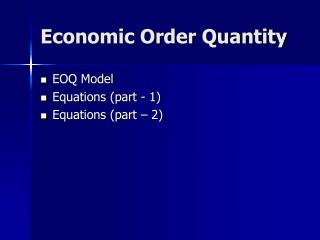DownloadDownload PresentationEconomic Order Quantity

# Economic Order Quantity

Télécharger la présentation## Economic Order Quantity

- - - - - - - - - - - - - - - - - - - - - - - - - - - E N D - - - - - - - - - - - - - - - - - - - - - - - - - - -
##### Presentation Transcript

1. Economic Order Quantity • EOQ Model • Equations (part - 1) • Equations (part – 2)

2. EOQ Model • Total Inventory cost is the minimum. • Annual demand of the item is constant and known. • Annual demand of the item is uniformly distributed through out the year. • Lead time is zero. • Total OC (P) = Total CC (R)

3. Equations For EOQ, Total OC (P) = Total CC (R) Total OC (p) = AO/Q Total CC (R) = QC/2 AO/Q = QC/2 Where, A = Annual Demand O = Ordering Cost ( Rs) / Order Q = EOQ C = Carrying Cost ( Rs )/ Year

4. Equations (part - 1) • Average Inventory = (Max. Inventory + Min. Inventory) / 2 Also, Average Inventory = Q / 2 • Total Inventory Cost (T) = P + R • Number of Orders per year = A/Q • Duration of one inventory cycle = Q/A

5. Problem Solution Zen Bicycles Ltd sources 3,000 seat covers for its bicycles from an outside supplier. The OC is Rs 10 per order and the CC is Rs 6 per unit per year. The company has 300 working days per year. Find • EOQ • Number of orders/year • Total Inventory Cost • Number of Inventory Cycles in a Year • Duration of an Inventory Cycle

6. Problem Solution The demand of small electric motor used in a fan is 20,000 per year. The price of the motor is Rs.100 per motor. The carrying cost is 8 percent of the purchase price. The ordering cost is Rs.200 per order. The number of working days is 320. • EOQ • Number of orders/year • Total Inventory Cost • Number of Inventory Cycles in a Year • Duration of an Inventory Cycle

7. Equation (Part – 2) EOQ model with shortages 2 • Q = (2AO/C)(C+K)/K Where Q = EOQ A = Annual Demand O = Order Cost C = Carrying Cost K = Cost of Shortage

8. Equation (Part – 2) Maximum inventory with shortages 2 • Q1 = (2AO/C)(K/(C+K) ) or = Q - S Where Q = EOQ A = Annual Demand O = Order Cost C = Carrying Cost K = Cost of Shortage S = Maximum Shortage

9. Equation (Part – 2) Shortage, S = CQ/(C+K) Where S = Maximum shortage Q = EOQ C = Carrying Cost K = Cost of Shortage

10. Equation (Part – 2) Maximum inventory = Q – S Reorder level = DDLT - S Where S = Maximum shortage Q = EOQ DDLT = Demand During lead time

11. Problem Vishal Computer sales (P) Ltd is a leading dealer of computer servers and net working devices at Raipur. Servers are expensive machines and therefore, Vishal follows a back ordering policy. The CC is Rs. 50,000 per servers per year and the OC is Rs 1,200 per order. The cost of shortage per server is estimated at Rs. 20,000. The annual demand of servers is 300 units. Find • The optimal order quantity (EOQ with intentional shortages) • Maximum shortage level • Maximum Inventory level • Reorder level, considering lead time 7 days and DDLT is 3 units.

12. Problem The annual demand for an automobile component is 24,000 units. The carrying cost is Rs 0.40/unit/year, the ordering cost is Rs.20,00 per order and the shortage cost is Rs.10./unit/year. Find • The optimal order quantity (EOQ with intentional shortages) • Maximum shortage level • Cycle Time • Maximum Inventory level • Reorder level, considering lead time 7 days and DDLT is 5 units.